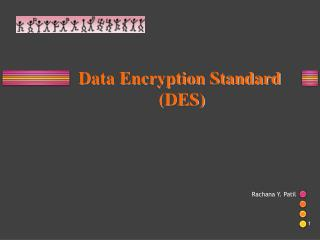DownloadDownload PresentationRachana Y. Patil

# Rachana Y. Patil

Download Presentation## Rachana Y. Patil

- - - - - - - - - - - - - - - - - - - - - - - - - - - E N D - - - - - - - - - - - - - - - - - - - - - - - - - - -
##### Presentation Transcript

1. Data Encryption Standard (DES) Rachana Y. Patil 1

2. INTRODUCTION The Data Encryption Standard (DES) is a symmetric-key block cipher published by the National Institute of Standards and Technology (NIST). 6.2

3. Overview DES is a block cipher, as shown in Figure Encryption and decryption with DES

4. DES STRUCTURE The encryption process is made of two permutations (P-boxes), which we call initial and final permutations, and sixteen Feistel rounds.

5. Initial and Final Permutations Initial and final permutation steps in DES

6. Note The initial and final permutations are straight P-boxes that are inverses of each other. They have no cryptography significance in DES.

7. Rounds DES uses 16 rounds. Each round of DES is a Feistel cipher. A round in DES (encryption site)

8. DES Function The heart of DES is the DES function. The DES function applies a 48-bit key to the rightmost 32 bits to produce a 32-bit output.

9. Expansion P-box Since RI−1 is a 32-bit input and KI is a 48-bit key, we first need to expand RI−1 to 48 bits.

10. Whitener (XOR) After the expansion permutation, DES uses the XOR operation on the expanded right section and the round key. Note that both the right section and the key are 48-bits in length. Also note that the round key is used only in this operation.

11. S-Boxes The S-boxes do the real mixing (confusion). DES uses 8 S-boxes, each with a 6-bit input and a 4-bit output. See Figure 6.7.

12. S-box rule

13. Cipher and Reverse Cipher Note Using mixers and swappers, we can create the cipher and reverse cipher, each having 16 rounds. First Approach To achieve this goal, one approach is to make the last round (round 16) different from the others; it has only a mixer and no swapper. In the first approach, there is no swapper in the last round.

14. DES cipher and reverse cipher for the first approach

15. Alternative Approach We can make all 16 rounds the same by including one swapper to the 16th round and add an extra swapper after that (two swappers cancel the effect of each other).

16. Key Generation The round-key generator creates sixteen 48-bit keys out of a 56-bit cipher key.

17. Parity-bit drop table Number of bits shifts

18. Strength of DES Two desired properties of a block cipher are • Avalanche effect • completeness.

19. Avalanche effect A small change in plaintext should create a significant change in ciphertext Example To check the avalanche effect in DES, let us encrypt two plaintext blocks (with the same key) that differ only in one bit and observe the differences in the number of bits in each round.

20. Although the two plaintext blocks differ only in the rightmost bit, the ciphertext blocks differ in 29 bits. This means that changing approximately 1.5 percent of the plaintext creates a change of approximately 45 percent in the ciphertext. Number of bit differences for Example

21. Completeness effect Completeness effect means that each bit of the ciphertext needs to depend on many bits on the plaintext. Confusionrefers to making the relationship between the key and the ciphertext as complex and involved as possible;  Diffusion means that the output bits should depend on the input bits in a very complex way. In a cipher with good diffusion, if one bit of the plaintext is changed, then the ciphertext should change completely, in an unpredictable  manner.

22. DES Weaknesses During the last few years critics have found some weaknesses in DES. Weaknesses in Cipher Design 1. Weaknesses in S-boxes 2. Weaknesses in P-boxes 3. Weaknesses in Key

23. Let us try the first weak key in Table 6.18 to encrypt a block two times. After two encryptions with the same key the original plaintext block is created. Note that we have used the encryption algorithm two times, not one encryption followed by another decryption. 6.23

24. Double encryption and decryption with a weak key

25. A pair of semi-weak keys in encryption and decryption

26. Double DES

27. Triple DES